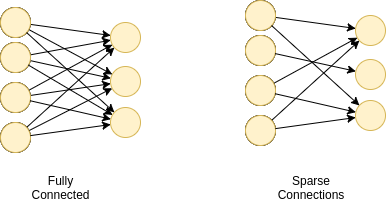# Custom connections in neural network layers

At the moment, I’m experimenting with defining custom sparse connections between two fully connected layers of a neural network.To accomplish this, right now I’m modifying `nn.Linear(in_features, out_features)` to `nn.MaskedLinear(in_features, out_features, mask)`, where mask is the adjacency matrix of the graph containing the two layers. The module `nn.Linear` uses a method invoked as `self._backend.Linear()` defined in `nn._functions.linear.py`, which I clearly will have to modify as well.

I’m not sure if this is the right way to do it. I have a constant feeling that there should be a better way to do this. Any help/comments on this are are much appreciated,

3 Likes

If you’re happy using just normal `nn.Linear`, then you can always zero out the weight matrix for the connections you want to remove. If they are zero then no information can go forwards or backwards through it (and hence the weights won’t change from zero), achieving the sparse connectivity that you want.

EDIT: This is wrong. See my last comment for more details.

Sorry but I don’t get your point. Do you mean to say that I should zero out the required weights before each forward phase?

That would definitely work for now but I was thinking if there was a more efficient solution.

EDIT: You’re right that no information will flow through those weights, but the weights themselves could change due to gradient descent right?

Just once at the beginning is enough. No gradients will flow through either, hence the weights shouldn’t change (unless you’re manually adjusting the weights in some other way).

I think you’ve got it wrong over here. Weights being zero at the beginning doesn’t guarantee weights being zero throughout.

If you have a mask already then you could do an element-wise multiply between the mask and the weights for every forward I would think? I don’t imagine an element-wise multiply would be too slow to do every forward. Alternatively, you could do something like this:

``````def zero_grad(self, grad_input, grad_output):

self.linear = nn.Linear(in_features, out_features)
self.linear.weights *= mask  # to zero it out first
``````

Then that way you can still have a fast forward with minimal overhead on the backward?

4 Likes

@Kaixhin I don’t understand the notation in the answer. I’m certain that the author either misinterpreted the question or got it wrong. If the derivative of a loss wrt a weight at zero is zero, then it would imply that assigning all weights to zero would lead to a stationary point, which is absurd.

Please note that I’m only assigning a subset of weights to zero and not all of them. I’m certain that what you claimed isn’t true and one needs to zero out the required weights for each forward pass.

Apologies, I was wrong. For a simple example with scalars, `y = w * x`,
even if `w = 0`, `dy/dw = x`, so the weights can indeed change from 0. I
agree with @bzcheeseman, masking in the forward pass seems like a
reasonable way to accomplish what you want.

2 Likes

@theQmech Can you comment if you were able to implement the mask based approach? And can you also say something about the speed-loss, if there was any?

Thank you.

As a dirty fix, I made a sub-class of nn.Linear. Initialize a 0-1 mask matrix, and multiply(elementwise) with weights with this mask during each backward pass. This implementation is no faster than a naive Linear transform. Note that this was in an older version of pytorch and I do not know if one can still achieve this.

I believe that pytorch0.2 has support for sparse matrix multiplication, which will make the computations much more efficient. Ideally, this is how it should be done.

@theQmech, I’m trying to implement the same exact thing but using sparse tensors for the weights as the full matrix won’t fit in memory. Do you have any hint on how to use sparse tensors and if cuSPARSE is supported? I made something with Theano but it doesn’t support multiple-CPU or GPU on sparse nets, so I’m looking to migrate to pytorch

@theQmech I am trying the same thing, do you have any progress?

I have tried to implement MaskedLinear.
↓↓↓

I am glad, if it help someone.

2 Likes

Here, also, is my implementation:

@uchida-takumi I would be interested in your thoughts on comparisons between the approaches.

1 Like

@mariob6 I did this a long time ago when sparse tensors were just proposed in PyTorch. Since then I haven’t played around with sparse tensors at all. Sorry can’t help you here.

You cant prevent the weights from changing during gradient descent. However, you can introduce a parameter called mask, which multiplies a mask with the weights, and use that to do the forward pass and the backward pass.
After this, you can use m.mask.shape, etc,. to look at the profile of the masked weights in your sparse network.
Here is my implementation:

``````class newlinear(Function):
super(newlinear, self).__init__()

#extendWeights is the masked weight which is used in the forward and backprop.
#Hence, the sparsity is maintained during backprop
def forward(self, input, weight):
self.save_for_backward(input, weight)
extendWeights = weight.clone()
output = input.mm(extendWeights.t())
return output

input, weight = self.saved_tensors
extendWeights = weight.clone()

###############################################################

class NewLinear(torch.nn.Module):
def __init__(self, input_features, output_features,matrix, mode='random'):
super(NewLinear, self).__init__()
self.input_features = input_features
self.output_features = output_features
self.matrix = matrix

kk += self.input_features

nn.init.kaiming_normal(self.weight.data,mode='fan_in')
'''Declaring the mask as a parameter'''

def forward(self, input):
``````
1 Like

Note for anyone trying to do this: If you use an optimiser with momentum e.g. Adam, then you will see gradient on each weight regardless of whether you set the weight to 0. You have to mask the actual gradient matrix before stepping your model.

Thank you so much for your contribution!

The novel Prune module also works for this.

``````import torch.nn.utils.prune as prune

# define some linear layer
out_features = 5
in_features = 10
linLayer = torch.nn.Linear(in_features, out_features, bias = False)